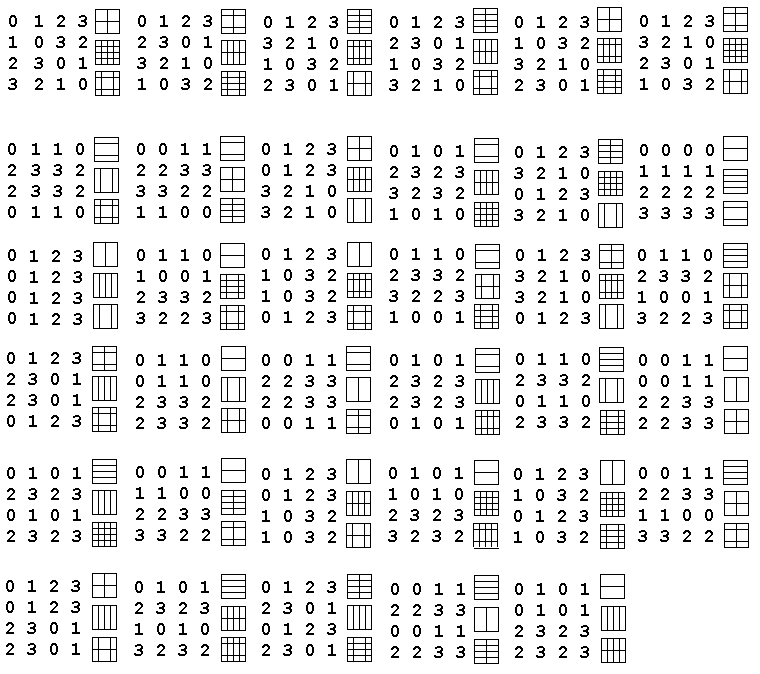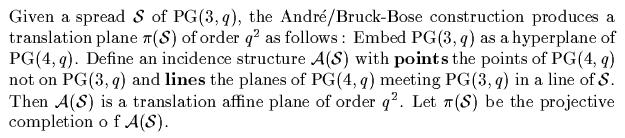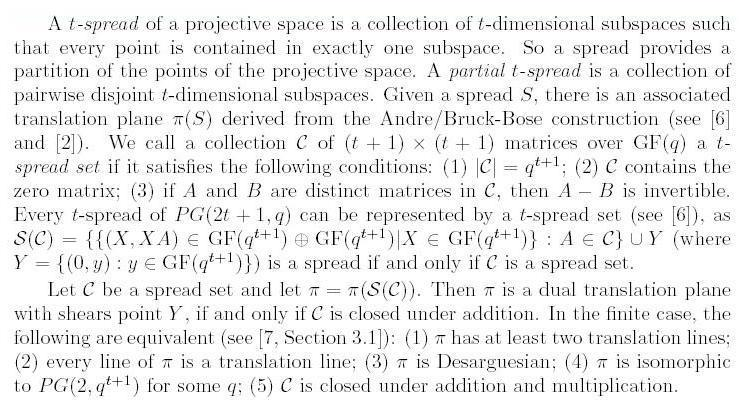Finite Geometry Notes   | Home | Site Map | Author |

Latin-Square Geometry:
Orthogonal Latin Squares as Skew Lines

#### by Steven H. Cullinane

"While the reader may draw many a moral from our tale,
I hope that the story is of interest for its own sake.
Moreover, I hope that it may inspire others, participants
or observers, to preserve the true and complete record of
our mathematical times."

-- From Error-Correcting Codes
Through Sphere Packings
To Simple Groups
,
by Thomas M. Thompson,
Mathematical Association of America, 1983

"Packing finite projective spaces with disjoint subspaces has for many years been a topic of considerable interest in Galois Geometry. In particular, one studies partial spreads in a space PG(3,q), that is, collections of pairwise disjoint lines in PG(3,q): see Hirschfeld  for background. A set of r mutually skew lines for which any other line meets at least one line of the set will be referred to as a maximal partial spread (MPS) of size r.

An interesting combinatorial problem (which seems at first sight not at all related to partial spreads) is the determination of the pairs (s,t) for which a maximal set of t mutually orthogonal Latin squares of order s exist...."

-- Some new maximal sets of mutually orthogonal Latin squares,
by P. Govaerts, D. Jungnickel, L. Storme, and J. A. Thas,
Designs, Codes, and Cryptography
29 (1-3), May-June-July 2003, pp. 141-147

Keywords: latin squares, MAXMOLS, partial spread, projective space, polar space

Mathematics Subject Classification: 05B15, 05B25, 05B40

These authors said, in early 2003, that spreads and orthogonal Latin squares seem "at first sight" unrelated. They of course did not mention my note (shown below) that pointed out such a relationship in December 1978. The earliest sources they cited for such a relationship are Jungnickel in 1984 and 1993.

Here are the details of my 1978 note.

We present two results -- one old, one new -- on the geometry of Latin squares.

### Result A (old):

There exist n-1 mutually orthogonal nxn Latin squares if and only if there exists a finite projective plane with n2 + n + 1 lines (or, equivalently, an affine plane with n2 + n lines).

Result A is well known. See, for instance, chapter 8 of Discrete Mathematics Using Latin Squares, C. F. Laywine and G. L. Mullen, Wiley Interscience, 1998, or Bose's  Theorem (pdf).

### Result B (new):

The six 4x4 Latin squares that have orthogonal Latin mates can be embedded in a set of thirty-five 4x4 arrays so that orthogonality in the set of arrays corresponds to skewness in the set of 35 lines of the finite projective space PG(3,2).

Result B is apparently new, and should not be confused with result A. The closest thing to the diagrams of result B in the refereed literature seems to be the use of diagrams on page 774 of Design Theory, Volume 2, by T. Beth, D. Jungnickel, and H. Lenz, Cambridge U. Press, 1999, in proving Tarry's theorem on the nonexistence of two mutually orthogonal 6x6 Latin squares.

The research note below shows how result B works. Note particularly that the 35-line projective space of result B differs from the 21-line projective plane of result A.

Result B is, of course, highly special, being limited to 4x4 squares. This limitation should not prevent its use as an example in popular introductions to discrete mathematics. Indeed, the 4x4 case figured prominently (and exclusively) on the cover and in a two-page article in the August/September 2001 issue of the Mathematical Association of America's "Focus" newsletter.

Naturally, a more general result than B is desirable; hence the problem stated in the 1978 research note below.

Steven H. Cullinane
Orthogonality of Latin squares viewed as skewness of lines.  Dec. 1978.

Shown below is a way to embed the six order-4 Latin squares that have orthogonal Latin mates in a set of 35 arrays so that  orthogonality in the set of arrays corresponds to skewness in the set of 35 lines of PG(3,2).  Each array yields a 3-set of diagrams that show the lines separating complementary 2-subsets of {0,1,2,3}; each diagram is the symmetric difference of the other two.  The 3-sets of diagrams correspond to the lines of PG(3,2).  Two arrays are orthogonal iff their 3-sets of diagrams are disjoint, i.e. iff the corresponding lines of PG(3,2) are skew.

This is a new way of viewing orthogonality of Latin squares, quite different from their relationship to projective planes.

PROBLEM:  To what extent can this result be generalized?View original 1978 note.

For related material, see the web pages
Geometry of the 4x4 Square and Diamond Theory.

The apparent conflict between the 2003 paper by Jungnickel et al. and my 1978 note can be resolved as follows:

"The [1954-1964] André/Bruck-Bose construction yields a one-to-one correspondence between spreads of projective space and translation planes (special affine planes). If one feeds a partial spread into this construction, a net results. A net is a point-line geometry which is a natural weakening of an affine plane."

And it has long been known that affine planes are, as noted above, closely related to orthogonal Latin squares.

In other words, the 1954-1964 André/Bruck-Bose construction is the missing (missing, that is, according to Jungnickel, Thas, et al.) link between Latin-square orthogonality and projective-space skewness. Such an orthogonality-skewness link is shown rather more directly and clearly in my 1978 note.

(For details of the André/Bruck-Bose construction, see

Johannes André, Über nicht-Dessarguessche Ebenen mit transitiver Translationsgruppe, Math Z. 60, pp. 156-186, 1954, and

R. H. Bruck and R. C. Bose, The construction of translation planes from projective spaces, J. Algebra 1, pp. 85-102, 1964.

The following may also be helpful:-- From Flocks, ovals, and generalized quadrangles (ps), (Four lectures in Napoli, June 2000), by Maska Law and Tim Penttila)

For further background, here is material on finite geometry from the paper Symplectic spreads (pdf), 15 Sept. 2003, by Simeon Ball, John Bamberg, Michel Lavrauw, and Tim Penttila:

"First we give an overview of some definitions and theory of finite geometry, together with some results of the past which provide the context and background for our construction.

A projective plane is an incidence structure of points and lines such that:

(PP1) for every pair of distinct points there is a unique line which is incident with both of them;

(PP2) every pair of distinct lines meet in a unique point;

(PP3) there exist four distinct points with no three collinear (no three are incident with a common line).

An affine plane is an incidence structure of points and lines such that:

(AP1) for every pair of distinct points there is a unique line which is incident with both of them;

(AP2) for any non-incident point-line pair p, L, there exists a unique line through p which has no point in common with L;

(AP3) there exist three non-collinear points.

In a projective or affine plane, a point P is a centre for a collineation phi if phi fixes every line incident with P.

A line l is an axis of phi if phi fixes every point on l. It is standard knowledge that every non-identity collineation has at most one axis and at most one centre, and it has an axis if and only if it has a centre (see [7, Section 3.1.4]). A collineation which has a centre and axis which are incident with one another, is called an elation. A group of collineations H is called (P, l)-transitive if the subgroup of H consisting of those elements which have centre P and axis l, acts transitively on the non-fixed points of any line through P which is not equal to l. For two lines m and l, we say that H is (m, l)-transitive if H is (P, l)-transitive for all P on m. Dually, if P and Q are points, then we say that H is (P, Q)-transitive if H is (P, l)-transitive for every line l incident with Q. Let Gamma be a projective plane and suppose that Delta is an affine plane obtained by removing the line linfinity from Gamma. Then Delta is a translation plane if there exists a (linfinity, linfinity)-transitive group of elations of Delta. We call linfinity the translation line of Delta. The dual of a translation plane is a shears plane, the corresponding point being a shears point..........

R. H. Bruck in 1951  introduced finite nets. A net is a system of points and lines satisfying:
(AP2) as before;
(N1) every two points lie on at most one line;
(N2) every point lies on at least two distinct lines.
Note that the definition of a net is the natural weakening of the axioms of an affine plane. Parallelism is an equivalence relation, and in the finite case, the number of parallel classes k is called the degree of the net, and the common number n of points on each line is called the order of the net. A net is thus equivalent to k - 2 mutually orthogonal n × n Latin squares, as shown by Bruck in 1951. See also Bruck's 1963 paper  for more on nets. There is an analogue here with the André/Bruck-Bose construction -- given a partial spread S, one can construct a net nu(S), but this time the converse fails."

 A. A. Albert. On the collineation groups associated with twisted fields. In Calcutta Math. Soc. Golden Jubilee Commemoration Vol. (1958/ 59), Part II, pages 485-497. Calcutta Math. Soc., Calcutta, 1958/1959.

 Johannes André. Über nicht-Desarguessche Ebenen mit transitiver Translationsgruppe. Math. Z., 60: 156-186, 1954.

 L. Bader, W. M. Kantor, and G. Lunardon. Symplectic spreads from twisted fields. Boll. Un. Mat. Ital. A(7), 8(3): 383-389, 1994.

 R. H. Bruck. Finite nets. I. Numerical invariants. Canadian J. Math., 3: 94-107, 1951.

 R. H. Bruck. Finite nets. II. Uniqueness and imbedding. Pacific J. Math., 13: 421-457, 1963.

 R. H. Bruck and R. C. Bose. The construction of translation planes from projective spaces. J. Algebra, 1: 85-102, 1964.

 P. Dembowski. Finite geometries. Ergebnisse der Mathematik und ihrer Grenzgebiete, Band 44. Springer-Verlag, Berlin, 1968.

 U. Dempwolff. Translation planes of order 27. Des. Codes Cryptogr., 4(2): 105-121, 1994.

 Christoph Hering. Eine nicht-desarguessche zweifach transitive affine Ebene der Ordnung 27. Abh. Math. Sem. Univ. Hamburg, 34: 203-208, 1969/ 1970.

 William M. Kantor. Commutative semifields and symplectic spreads. To appear in J. Algebra.

 William M. Kantor. Strongly regular graphs defined by spreads. Israel J. Math., 41(4): 298-312, 1982.

 T. G. Ostrom. Replaceable nets, net collineations, and net extensions. Canad. J. Math., 18: 666-672, 1966.

 Chihiro Suetake. A new class of translation planes of order q3. Osaka J. Math., 22(4): 773-786, 1985.

 Fam Khyu T'ep. Irreducible J-decompositions of the Lie algebras Apn- 1. Mat. Zametki, 49(5): 128-134, 159, 1991.

 J. A. Thas. Ovoids and spreads of finite classical polar spaces. Geom. Dedicata, 10(1-4): 135-143, 1981.

 J. A. Thas. Old and new results on spreads and ovoids of finite classical polar spaces. In Combinatorics '90 (Gaeta, 1990), volume 52 of Ann. Discrete Math., pages 529-544. North-Holland, Amsterdam, 1992.

 J. A. Thas. Ovoids, spreads and m-systems of finite classical polar spaces. In Surveys in combinatorics, 2001 (Sussex), volume 288 of London Math. Soc. Lecture Note Ser., pages 241-267. Cambridge Univ. Press, Cambridge, 2001.

Further sources of material on
latin-square orthogonality
and projective skewness

For the use of the term "partial spreads" to mean sets of affine parallel classes (as interpreted within graph theory), see

(ps),
a note by Chris Godsil:

"Let Z be a complete graph on n2 vertices. A parallel class in Z is a spanning subgraph isomorphic to nKn.
We say two parallel classes S1 and S2 are orthogonal if they have no edges in common....
A partial spread is a set of pairwise orthogonal parallel classes."

This informal note has no references.  The way the note uses the terms "partial spread" and "orthogonal" is directly related to my 1978 note.  Historians of mathematics can determine whether Godsil is the first to use these terms with these meanings.

Background for Godsil's note:

Strongly Regular Graphs (ps),
by Peter J. Cameron (draft of a survey article), and
two references cited by Cameron:

• C. D. Godsil, Algebraic Combinatorics, Chapman & Hall/CRC Press, 1993, and
• R. C. Bose, Strongly regular graphs, partial geometries, and partially balanced designs, Pacific J. Math. 13 (1963), 389-419.